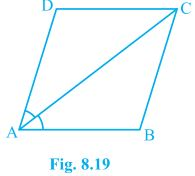# Diagonal AC of a parallelogram ABCD bisects ∠A (see Fig.) . Show that (i) it bisects ∠C also, (ii) ABCD is a rhombus.(i) it bisects ∠C also,

Since ABCD is a parallelogram.

∠BAC = ∠DCA ……………………………(1) [Alternate interior angles are equal]

∠CAD = ∠BCA ………………………….(2) [Alternate interior angles are equal]

Also, ∠CAD = ∠CAB …………………….(3) [ (Given) as AC bisects ∠A]

From (1), (2) and (3), we have

∠DCA = ∠BCA

Hence, AC bisects ∠C.

(ii) ABCD is a rhombus.

In ∆ABC,

∠BAC = ∠DCA ………………………….. [Alternate interior angles are equal]

BC = AB …………………………….(4) [ Sides opposite to equal angles of a ∆ are equal]

But, ABCD is a parallelogram. [Given]

AB = DC ………………….(6) (opposite sides of parallelogram)

From (4), (5) and (6), we have

AB = BC = CD = DA

As, ABCD is a parallelogram having all sides equal then it is a rhombus.